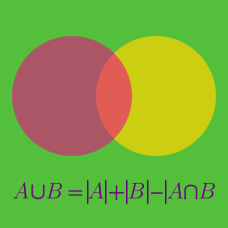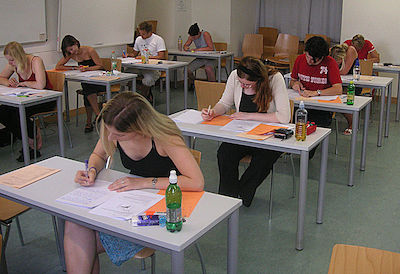Probability

# Principle of Inclusion and Exclusion Problem SolvingAfter a grueling nationwide examination, it was announced that
70% of students passed the Mathematics examination, and
60% of students passes the Science examination.

What is the minimum percentage of students that passed both examinations?

In a class of students, $64\text{\%}$ of the students passed the physics exam and $68\text{\%}$ of the students passed the math exam.

What is the minimum percentage of students in the class that passed both exams?

How many numbers in the set $\{1,2,\ldots,100\}$ are divisible by $5$ or $7?$

A party of $370$ vile creatures is marching through the woods. Each of the creatures is either an orc, a goblin, or a half-orc/half-goblin. If there are $206$ creatures that are at least partly goblin, and $211$ creatures that are at least partly orcs, how many of the creatures are half-orc/half-goblins?

Consider all $4$-digit positive numbers using all of the digits $\{1, 2, 3, 4\}$. How many of these numbers have even first or second digit?

×